# Why #NA occur And How to Fix #NA in Excel

In this article, we will be learning why #N/A occur And How to Fix #N/A in Excel.

In excel, at times we get #N/A error. This error occurs when there is something important missing with something which can't be found or identified in the formula you have supplied to the cell and is recognized by Excel by showing the error. As per Microsoft official site a #N/A is Excel's way of saying, the value you are looking for is not available in the list you provided.

If you are looking for some value in the table and if it can't be found or identified, this error suggests you to check some points discussed below.

Let’s understand how this error occurred and how to fix them with some examples

The most basic reason of #N/A error is when cell value is not found in the list. Generally Excel matches the value with each element of the lookup array and returns the first matched value if found or else it returns #N/A error. Here #N/A error suggests that Value you are looking for in the list is not found. See the below example to understand more.

For example, LOOKUP function matches the value in the first array and returns value from the corresponding second array. In the data shown below.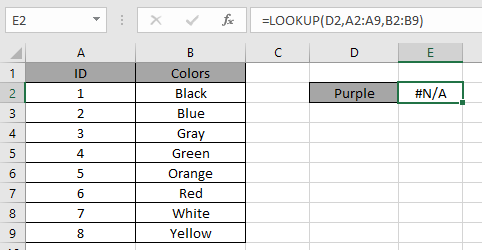Look in the above snapshot and realize the mistake just happened. The above function looks for the value "Purple" in the ID column which obviously it won't be able to find.

How to fix it! You cannot fix the error for the result if there's no matched value in the result. But the first problem here is that we are looking at the wrong array. Check the array that you are giving as an argument to the function.

two ways to catch this error which returns the #N/A Error

First method is via Using IFERROR function to catch the error by the function

Generic formula:

 = IFERROR ( FUNCTION , "response" )

Explanation:

• The function performs its task as it is supposed to perform.
• IFERROR function returns the value if found.
• But IFERROR function returns the "response" if any error is found.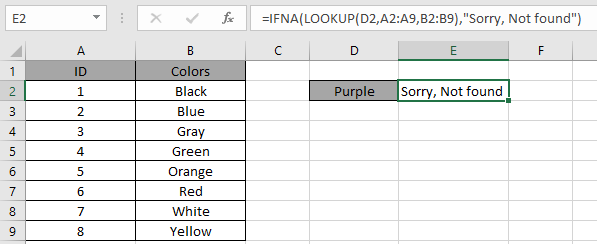The second method is via the IFNA function to catch the error by the function. This function is exclusively made to catch the #N/A error.

Generic formula:

 = IFNA ( FUNCTION , "response" )

Explanation:

• The function performs its task as it is supposed to perform.
• IFNA function returns the value if found.
• IFNA function returns the "response" if any error is found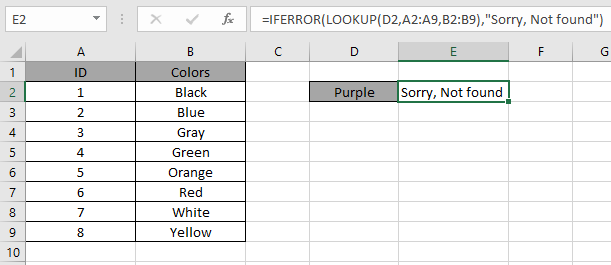As you can see the #N/A Error can be handled when operating with functions.
#N/A error due value not identified

The most basic reason of #N/A error is when cell value not identified in the list. Generally Excel matches the value with each element of the lookup array and returns the first matched value if found or else it returns #N/A error. Here #N/A error suggests that Value you are looking in the list is not identified. See the below example to understand more.

For example, LOOKUP function matches the value in the first array and returns value from the corresponding second array. In the data shown below.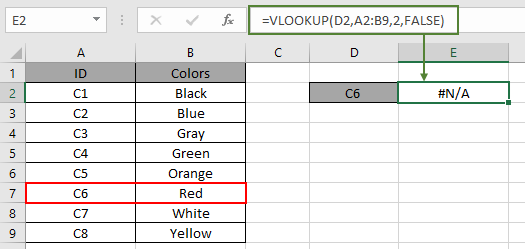Look in the above snapshot and realize the mistake just happened. The above function looks for the ID "C6" in the ID column which is marked in RED box. So why the function is not giving the output.

How to fix it! You can fix this error for the result, follow the points shown below.

1. The lookup value is spelled correctly and does not contain extra space characters.
2. Values in the lookup table are spelled correctly and do not contain extra space.
3. The lookup table contains all required values.
4. The lookup range provided to the function is complete.
5. Lookup value type = lookup table type (i.e. both are text, both are numbers, etc.)
6. Matching (approximate vs. exact) is set correctly.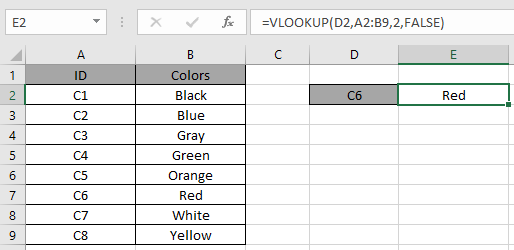In the above snapshot there was an error with extra space.

As you can see the #N/A Error can be handled when operating with functions.

## Trapping #N/A error and no message "response"

two ways to catch this error which returns the #N/A Error

First method is via Using IFERROR function to catch the error by the function

Generic formula:

 = IFERROR ( FUNCTION , "" )

Explanation:

• The function performs its task as it is supposed to perform.
• IFERROR function returns the value if found.
• But IFERROR function returns the empty string ( "" ) if any error is found.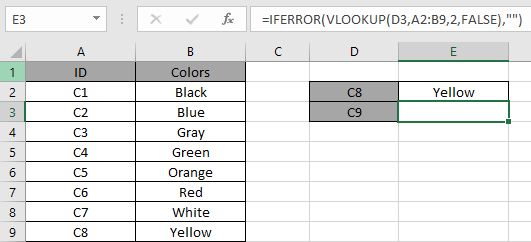In the above snapshot there was an error with extra space.

As you can see the #N/A Error can be handled when operating with functions.

## Trapping #N/A error and no message "response"

two ways to catch this error which returns the #N/A Error

First method is via Using IFNA function to catch the error by the function
Generic formula:

 = IFNA ( FUNCTION , "" )

Explanation:

• The function performs its task as it is supposed to perform.
• IFNA function returns the value if found.
• But IFNA function returns the empty string ( "" ) if any error is found.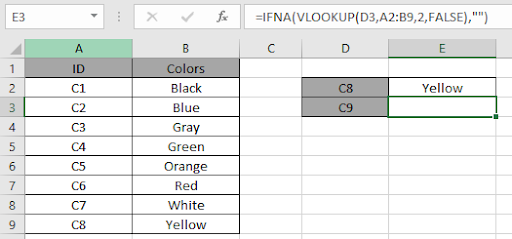In the above snapshot the function returns the empty string ( "" ) as response instead of the error.

Trapping #N/A error with INDEX and MATCH function

IF using the INDEX and MATCH function instead of VLOOKUP function. See the below snapshot and the formula used in the table. Use the formula.

 = IFERROR ( INDEX ( B2:B9 , MATCH ( D3 , A2:A9 , 0 ) ) , "Not found" )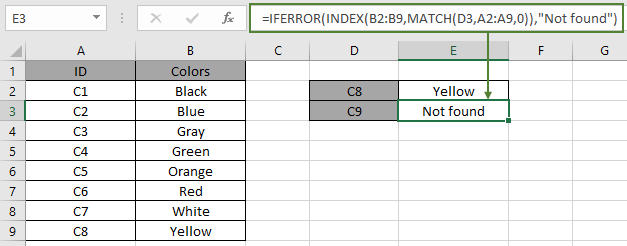As you can see how #N/A error with function returns #N/A error and after the correction the formula works fine.

In excel, you can force the #N/A error if no need to display as the result. Using the NA function of Excel.

Use the formula:

 = IF ( A3 = "" , NA() , "Non-blank" )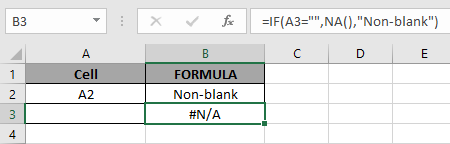Hope this article about how Why #N/A occur And How to Fix #N/A error in Excel is explanatory. Find more articles on Excel errors functions here. Please share your query below in the comment box. We will assist you.

Related Articles

#VALUE Error And How to Fix It in Excel

How to correct a #NUM! Error

How to use wildcards in excel

How to Remove Text in Excel Starting From a Position

Validation of text entries

Create drop down list in excel with colour

Remove leading and trailing spaces from text in Excel

Popular Articles

Edit a dropdown list

Absolute reference in Excel

If with conditional formatting

If with wildcards

Vlookup by date

Join first and last name in excel

Count cells which match either A or B

Convert Inches To Feet and Inches in Excel 2016

50 Excel Shortcut to Increase Your Productivity

Terms and Conditions of use

The applications/code on this site are distributed as is and without warranties or liability. In no event shall the owner of the copyrights, or the authors of the applications/code be liable for any loss of profit, any problems or any damage resulting from the use or evaluation of the applications/code.